# MB-Ruler Pro function overview

The most important facts:

#### The application

MB-Ruler Pro can measure distances, angels and areas in polygons, circles and ellipses.
The measure values can be exported by different ways for the use in other applications.

#### The application modes

MB-Ruler Pro can work in two different ways. All measure instruments, coordinate systems and functions are available in both modes.
 Window mode Using the window mode MB-Ruler Pro starts as a normal Windows application. The window mode is useful for measuring objects in images, from different data sources (photos, scans, ...). Overlay Mode Using the overlay mode you can use the measure instruments of MB-Ruler Pro transparent above other applications and integrate MB-Ruler Pro seamless in these applications. This mode is ideal to measure objects in application which have no or insufficient measure possibilities. When MB-Ruler Pro is started in overlay mode, you can toggle both modes.

#### The coordinate systems

MB-Ruler Pro can measure in four different coordinate systems: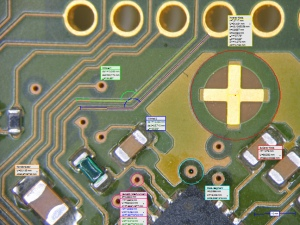Orthogonal projection The orthogonal projection is the most often used coordinate system. Use it, if the distance and unit in all directions is equal. (example)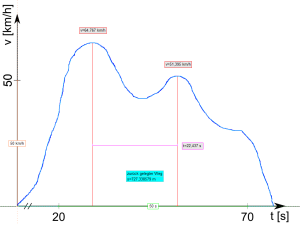X-Y Coordinate system Use the X-Y coordinate system to measure, if the scales and units of the x- and y-scales differ, p.e. when analysing and digitizing scientific diagrams. The X-Y coordinate system support linear, invers and logarithmic scales. (example)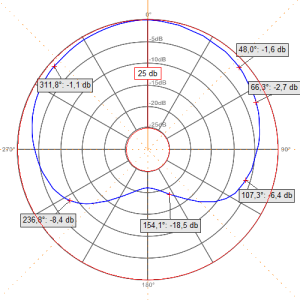Polar coordinate system Use the polar coordinate system to analyse and digitize p.e. diagrams in polar coordinates. The polar coordinate system support a linear and a logarithmic scales.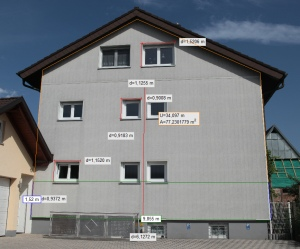Perspective projection Use the perspective projection to measure in photos with perspective distortion: the size of a wall the size of damaged areas on the street ...

#### The measure instruments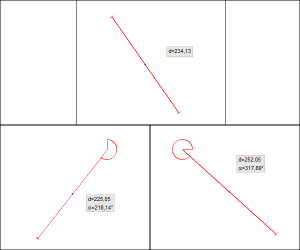Length of a line + angle to a horizontal or vertical line Measure the distance between to points and optional the angle between this line and a horizontal or vertical line. Angle origin and orientation can be selected.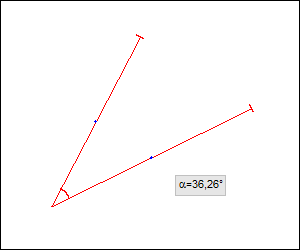Angle (intersection point in visible area) Measure an angle in the interval 0° bis 360° between two line. Their intersection point is on the screen or inside the image.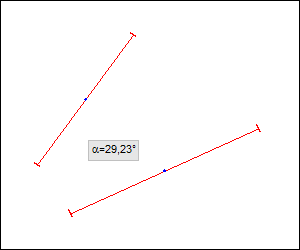Angle between two lines who do not intersect in the visible area Measure an angle in the interval 0° bis 360° between two lines, who do not intersect on the screen or inside the image. The lines are defined by two points on each line.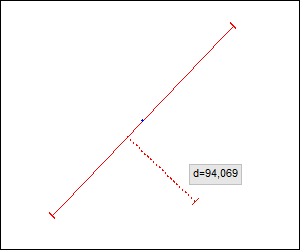Distance "point to line" Measure the shortest distance between a point and and line.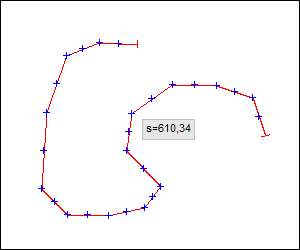Length of a polyline+distance "point to polyline" Measure the length of a polyline with up to 1000 points. Optional you can additionally measure the distance between a point and this polyline.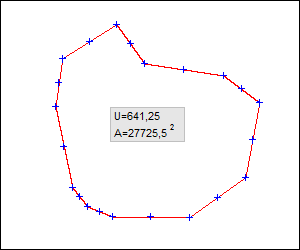Circumference and area of a polygon+distance "point to polygon" Measure the circumference and area of a polygon with up to 1000 points. Optional you can additionally measure the distance between a point and this polygon.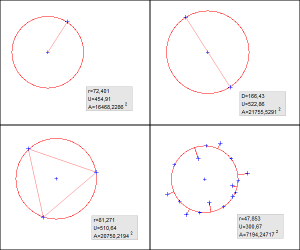Parameters of a circle - defined by center and point on the circle (radius) two diametral points on the circle (diameter) three points on the circle several points on the circle (interpolation circle) Measure the radius, diameter, circumference and area of a circle, independent of the available geometric information of the circle.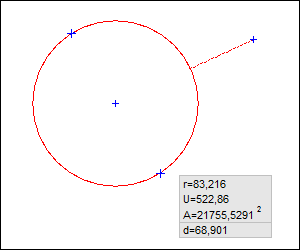Distance "point to circle" Measure the shortest distance between a point and a circle - independent of how the the circle was defined.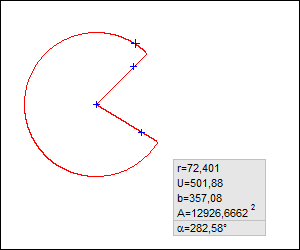Circular sector Measure the radius, circumference, length of the arc, area and angle of a circular sector - independent of how the the circle was defined.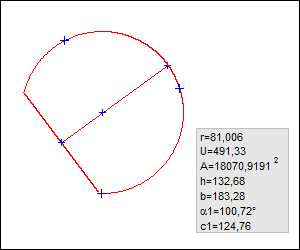Circular segment Measure the radius, circumference, length of the arc, length of the chord, area and angle of a circular segment - independent of how the the circle was defined. Optional you can crop the circle by two parallel lines.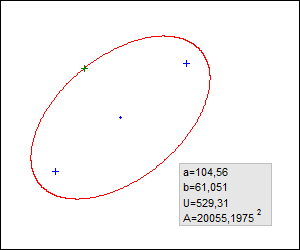Parameters of an ellipse+distance "point to ellipse" Measure the semi-major, semi-minor, the circumference and the area of an ellipse. The ellipse is defined by the its focal points and a point on the ellipse. Optional you can additionally measure the distance between a point and this ellipse.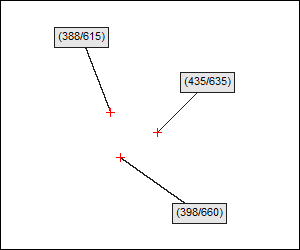Marker point Mark positions on the screen or in an image to show the coordinate of this point or add a description text to this point.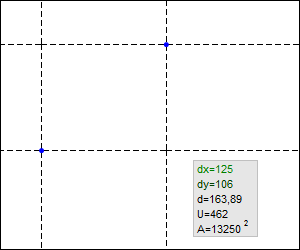Parameters of a rectangle (double cross hair) Measure the width, height, diagonal, circumference and rectangle of a rotatable rectangle.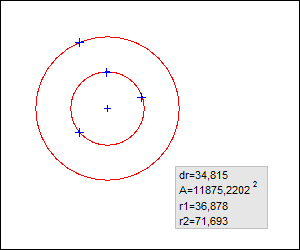Two concentric circles (circular ring) Measure the difference of the radiuses of two concentric circles and the area of a circular ring. The first circle is defined by three points on the circle and the second circle by one point on the circle.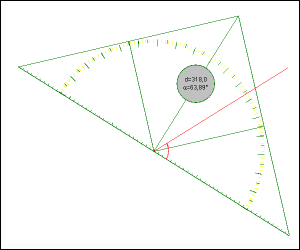... and: a set square with integrated protractor Measure distances and angles as you know it from measuring them with a real triangular ruler from school. User defined measure instruments Additional to the existing measure instruments, MB-Ruler Pro can handle user defined measure instruments and templates to exactly fit your use case. Complex combination of lines, circles and ellipses are possible.